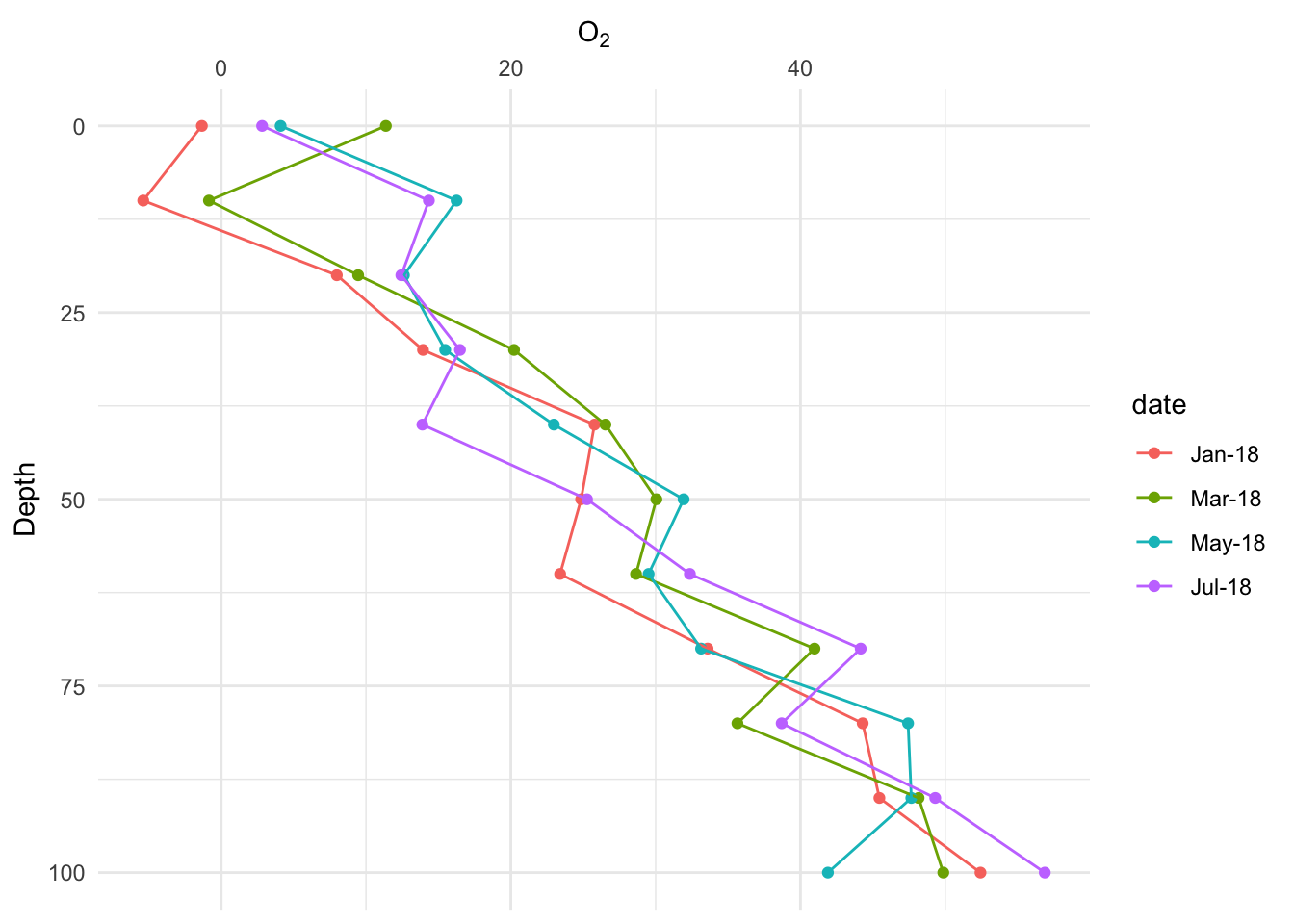set up

The goal is to plot the measure of something, say O2 levels, against depth (soil or lake), with the measures taken on multiple days

library(ggplot2)
library(data.table)

First – create fake data

depths <- c(0, seq(10,100, by=10))
dates <- c("Jan-18", "Mar-18", "May-18", "Jul-18")
x <- expand.grid(date=dates, depth=depths)
n <- nrow(x)
##     date depth
## 1 Jan-18     0
## 2 Mar-18     0
## 3 May-18     0
## 4 Jul-18     0
## 5 Jan-18    10
## 6 Mar-18    10
X <- model.matrix(formula(~date + depth), data=x)
##   (Intercept) dateMar-18 dateMay-18 dateJul-18 depth
## 1           1          0          0          0     0
## 2           1          1          0          0     0
## 3           1          0          1          0     0
## 4           1          0          0          1     0
## 5           1          0          0          0    10
## 6           1          1          0          0    10
beta <- c(0,1,2,3,0.5)
y <- X%*%beta + rnorm(n, sd=5)
fake_data <- data.frame(O2=y, x)
##           O2   date depth
## 1 -1.3329187 Jan-18     0
## 2 11.3715570 Mar-18     0
## 3  4.1045517 May-18     0
## 4  2.8264293 Jul-18     0
## 5 -5.3809814 Jan-18    10
## 6 -0.8433094 Mar-18    10

Second – make ggplot

gg <- ggplot(data=fake_data, aes(x=depth, y=O2, group=date, color=date)) +
geom_point() +
geom_line() +
coord_flip() +
# coord_flip does not make the y-axis the x-axis but the horizontal axis.
# so still need to refer to "y" to modify O2
# specify position="right" to flip the y axis to the top after coord_flip
scale_y_continuous(position = "right") +
# reverse the depth axis, which makes it go down instead of up
scale_x_reverse() +
xlab("Depth") +
ylab(expression(O)) +
theme_minimal() +
NULL
gg